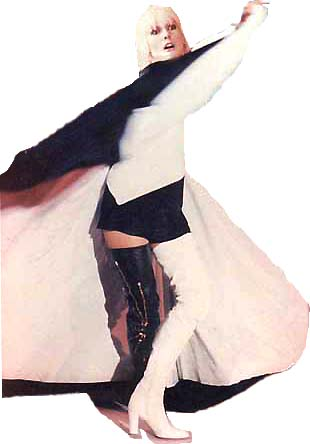## Practical C ProgrammingThe SplusD Lab is proud to announce a ‘Practical C Programming Workshop’

duration: 3 months

number of lesson: 24
starting: 5th February

monday – thursday h 21 – 23
door open at 20:30

reference text: Kernighan Ritchie “The C Programming Language”

damage: 60 euro (non compulsory) including exercises and photocopies (covering teacher’s travel expenses)
number of students = 6

Professor: Geeno Zeffirelli (Bakunio), writer and programmer, developer of Morla, Soma and much more

http://autistici.org/bakunin/

Every lesson will include exercises.

This course is divided in two parts. The first is the C syntax and the basics of programming.
The second part is about operating system, algorithms, threads, externall libraries and networking.

A two weeks break will be held from the 15th of March. Here the students will be invited to start working on a new application of their choice.

xname (at) squat (dot) net

Program:

MODULE_1

Lesson 1 = syntax 1 – intro, hello world – NotesExerciseSolution

Lesson 2 = syntax 2 – variables, cycles (for, while, if, switch) – ExerciseSolution1Solution2
Lesson 3 = syntax 3 – variables types, arrays – ExerciseSolution1Solution2
Lesson 4 = syntax 4 – pointers – ExerciseSolution1Solution2Solution3Solution4
Lesson 5 = syntax 5 – functions, variables scope – ExerciseSolution1Solution2
Lesson 6 = data struct – ExerciseSolution
Lesson 7 = libraries (mathematical, standard input / standard output)

Lesson 8 = files (open, close, create, remove)

Lesson 9 = style of an application / basic data structure (cues, lists)

Lesson 10 = basic algorithms (sorting, searching)

Lesson 11 = trees, matrix, graphs

Lesson 12 = overview, questions and homeworks

==== holidays

MODULE_2

Lesson 13 = homeworks correction *

Lesson 14 = posix (linux, bsd, minix, mac, 80% win), fork, mount / Operations with C kernel

Lesson 16 = mutua esclusione mutex, semafori

Lesson 17 = socket, tcpip, udp, server and client, network system call

Lesson 18 = client – server network applications / TCP/IP

Lesson 19 = external libraries usage / text editing /

Lesson 20 = graphical libraries

Lesson 21 = video libraries

Lesson 22 = testing / explanation / debugging of the applications developed by the students

Lesson 23 = testing / explanation / debugging of the applications developed by the students

Lesson 24 = net and suk